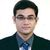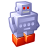# QlikView App Dev

Discussion Board for collaboration related to QlikView App Development.

cancel
Showing results for
Did you mean:Contributor II

## Using variables in a sum formula

I can not get this formula to work with a variable.

I want to replace the 6 with a variable (manually set month)

Can anyone help me?

Sum({<Month={'<=6'},Year={"\$(=Year(Today()))"},[Dept. No]={'820'},Project={'SKAND-820*'}>} Hours)

I have made a variable "vMonth" (see below) but it does not work.1 Solution

Accepted SolutionsMVP

Try this,

Sum({<Month={'<=\$(vMonth)'},Year={"\$(=Year(Today()))"},[Dept. No]={'820'},Project={'SKAND-820*'}>} Hours)

Regards,

Kaushik Solanki

Regards,
Kaushik
5 RepliesMVP

How did you try putting the variable in the expression? Like:

Sum({<Month={'<=\$(vMonth)'},... ?MVP

Try this,

Sum({<Month={'<=\$(vMonth)'},Year={"\$(=Year(Today()))"},[Dept. No]={'820'},Project={'SKAND-820*'}>} Hours)

Regards,

Kaushik Solanki

Regards,
KaushikContributor III

Sum({<Month={'<=\$(vMonth)'},Year={\$(=Year(Today()))},[Dept. No]={'820'},Project={"SKAND-820*"}>} Hours)Contributor II
Author

Thanks a LOT 😉Contributor II
Author

Works 🙂 Thanks a lot for your help.Community Browser